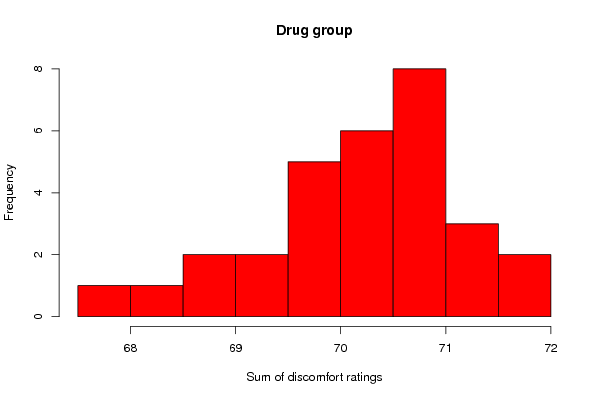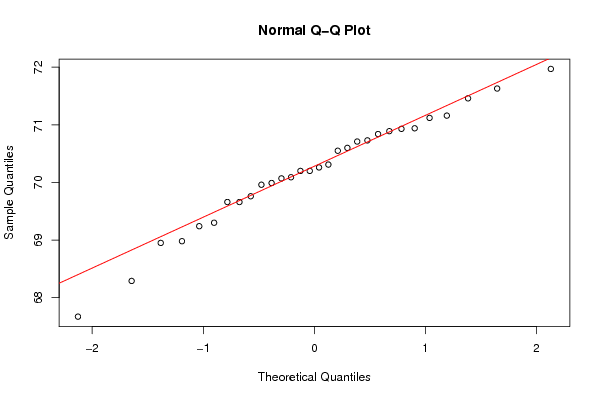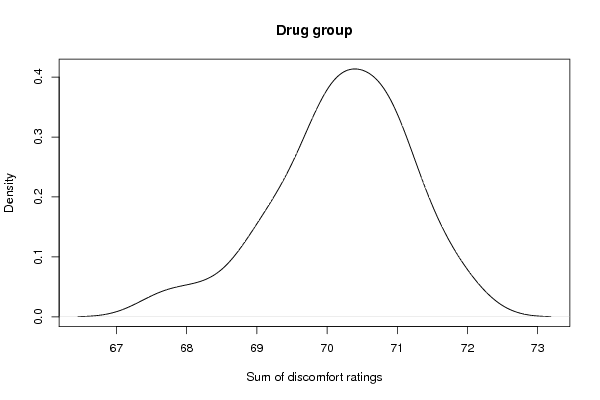## Free Statistics

of Irreproducible Research!

Author's title
Author*The author of this computation has been verified*
R Software Modulerwasp_Distributional Plots.wasp
Title produced by softwareHistogram, QQplot and Density
Date of computationFri, 11 Nov 2011 09:16:17 -0500
Cite this page as followsStatistical Computations at FreeStatistics.org, Office for Research Development and Education, URL https://freestatistics.org/blog/index.php?v=date/2011/Nov/11/t1321021004f1fonclhsx2w18u.htm/, Retrieved Fri, 02 Jun 2023 07:40:08 +0000
Statistical Computations at FreeStatistics.org, Office for Research Development and Education, URL https://freestatistics.org/blog/index.php?pk=141345, Retrieved Fri, 02 Jun 2023 07:40:08 +0000
QR Codes:Original text written by user:
IsPrivate?No (this computation is public)
User-defined keywords
Estimated Impact81
Family? (F = Feedback message, R = changed R code, M = changed R Module, P = changed Parameters, D = changed Data)
-     [Aston University Statistical Software] [Morning Sickness ...] [2009-11-16 16:26:06] [74be16979710d4c4e7c6647856088456]
- R     [Aston University Statistical Software] [Morning Sickness ...] [2009-11-16 17:22:16] [74be16979710d4c4e7c6647856088456]
-   P     [T-Tests] [Morning Sickness ...] [2010-11-09 11:12:43] [3fdd735c61ad38cbc9b3393dc997cdb7]
- RM        [T-Tests] [Morning Sickness ...] [2011-11-07 09:34:35] [98fd0e87c3eb04e0cc2efde01dbafab6]
- RMPD          [Histogram, QQplot and Density] [distributional pl...] [2011-11-11 14:16:17] [c3a1a4881a39c19cd8024386f5bb4813] [Current]
Feedback Forum

Post a new message
Dataseries X:
68.95
70.84
70.26
70.93
70.71
70.73
70.2
69.76
71.46
70.31
69.24
70.94
68.98
71.16
69.96
69.66
70.2
69.99
71.97
68.29
67.67
70.07
70.89
69.66
71.12
70.6
70.09
70.55
71.63
69.3

 Summary of computational transaction Raw Input view raw input (R code) Raw Output view raw output of R engine Computing time 2 seconds R Server 'George Udny Yule' @ yule.wessa.net

\begin{tabular}{lllllllll}
\hline
Summary of computational transaction \tabularnewline
Raw Input & view raw input (R code)  \tabularnewline
Raw Output & view raw output of R engine  \tabularnewline
Computing time & 2 seconds \tabularnewline
R Server & 'George Udny Yule' @ yule.wessa.net \tabularnewline
\hline
\end{tabular}
%Source: https://freestatistics.org/blog/index.php?pk=141345&T=0

[TABLE]
[ROW][C]Summary of computational transaction[/C][/ROW]
[ROW][C]Raw Input[/C][C]view raw input (R code) [/C][/ROW]
[ROW][C]Raw Output[/C][C]view raw output of R engine [/C][/ROW]
[ROW][C]Computing time[/C][C]2 seconds[/C][/ROW]
[ROW][C]R Server[/C][C]'George Udny Yule' @ yule.wessa.net[/C][/ROW]
[/TABLE]
Source: https://freestatistics.org/blog/index.php?pk=141345&T=0

Globally Unique Identifier (entire table): ba.freestatistics.org/blog/index.php?pk=141345&T=0

As an alternative you can also use a QR Code:

The GUIDs for individual cells are displayed in the table below:

 Summary of computational transaction Raw Input view raw input (R code) Raw Output view raw output of R engine Computing time 2 seconds R Server 'George Udny Yule' @ yule.wessa.net

 Descriptive Statistics mean 70.204 formula standard deviation 0.966282 formula

\begin{tabular}{lllllllll}
\hline
Descriptive Statistics \tabularnewline
mean & 70.204 & formula \tabularnewline
standard deviation & 0.966282 & formula \tabularnewline
\hline
\end{tabular}
%Source: https://freestatistics.org/blog/index.php?pk=141345&T=1

[TABLE]
[ROW][C]Descriptive Statistics[/C][/ROW]
[ROW][C]mean[/C][C]70.204[/C][C]formula[/C][/ROW]
[ROW][C]standard deviation[/C][C]0.966282[/C][C]formula[/C][/ROW]
[/TABLE]
Source: https://freestatistics.org/blog/index.php?pk=141345&T=1

Globally Unique Identifier (entire table): ba.freestatistics.org/blog/index.php?pk=141345&T=1

As an alternative you can also use a QR Code:

The GUIDs for individual cells are displayed in the table below:

 Descriptive Statistics mean 70.204 formula standard deviation 0.966282 formulaPNG link Postscript link PDF linkPNG link Postscript link PDF linkPNG link Postscript link PDF link

Parameters (Session):
par1 = 10 ;
Parameters (R input):
par1 = 10 ;
R code (references can be found in the software module):
bitmap(file='test1.png')par1 <- as.numeric(par1)myhist<-hist(x, breaks=par1, col=2, xlab=xlab, main=main)dev.off()bitmap(file='test2.png')qqnorm(x)qqline(x, col=2)dev.off()bitmap(file='test3.png')plot(density(x), xlab=xlab, main=main)dev.off()load(file='createtable')a<-table.start()a<-table.row.start(a)a<-table.element(a,'Descriptive Statistics',3,TRUE)a<-table.row.end(a)a<-table.row.start(a)a<-table.element(a,'mean',header=TRUE)a<-table.element(a,signif(mean(x), 6))a<-table.element(a,hyperlink('arithmetic_mean.htm','formula','click to see the formula'))a<-table.row.end(a)a<-table.row.start(a)a<-table.element(a,'standard deviation',header=TRUE)a<-table.element(a,signif(sd(x), 6))a<-table.element(a,hyperlink('unbiased1.htm','formula','click to see the formula'))a<-table.row.end(a)a<-table.end(a)table.save(a,file='mytable.tab')